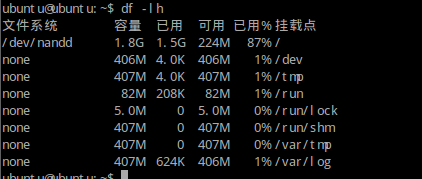# 如何打开pcDuino上的4GB的nand flash

pc朵拉 发布于 2014/01/07 17:20\$chmod +x pcduino-nand-4g-fix
\$ sudo ./pcduino-nand-4g-fix

partition  0: name =   bootloader, partition start =     2048, partition size =    65536
partition  1: name =          env, partition start =    67584, partition size =     4096
partition  2: name =         boot, partition start =    71680, partition size =    32768
partition  3: name =       rootfs, partition start =   104448, partition size =  3686400
partition  4: name =        UDISK, partition start =  3790848, partition size =  3991552
5 partitions
check partition table copy 0: OK
check partition table copy 1: OK
check partition table copy 2: OK
check partition table copy 3: OK
ready to write new partition tables:
partition  0: name =   bootloader, partition start =     2048, partition size =    65536
partition  1: name =          env, partition start =    67584, partition size =     4096
partition  2: name =         boot, partition start =    71680, partition size =    32768
partition  3: name =       rootfs, partition start =   104448, partition size =  7677952
4 partitions
write new partition tables? (Y/N)
y
输入y确认, 然后系统会自动重启生效.